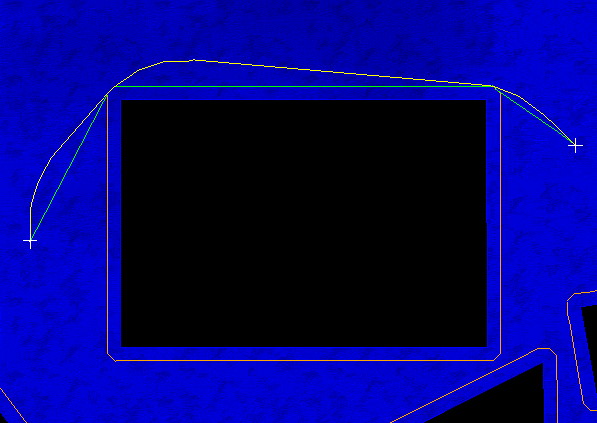PathEngine home previous: Aborting Pathfinding Early next: Advancing Along Paths
Contents, Programmers Guide, Applying the SDK, Path Post Processing

### Path Post Processing

The geometric shortest path for an agent in a polygon environment will consist of a sequence of straight line segments with sharp turns at corners.
If we are looking for smooth agent movement, then these sharp turns may not be desirable.
PathEngine solves this problem with path post processing.

iMesh::generateCurvedPath_WithEndVector() and iAgent::generateCurvedPath_WithEndVector() provide a way to smooth the corners of a path returned by one of the pathfinding queries.

Internally this method works by generating curves at corners and then testing for collision.
This means that the overhead for calling this method is relatively low.
In most situations (and depending on the parameters used) sharp corners will be successfully smoothed.
In pathological situations, where a given corner cannot be smoothed, the method falls back on the sections from the base path.
This way we maintain the same guarantees about movement to the destination as applied to the original path.

The resulting path will be a sequence of straight line sections to approximate a curve.
Curvature is controlled in terms of a desired turn angle and section length.

For each corner a curve is first attempted for 'turnRatio1' and 'sectionLength'.
If this collides then a second curve is attempted for 'turnRatio2' and 'sectionLength'.
(So 'turnRatio2' should be sharper than 'turnRatio1'.)
If the second curve fails then the corner is left as is.

A curve may pass a number of corners before rejoining the base path.

#### Turn ratios

Ratios are used instead of angles to avoid the need to call trigonometric functions.
The ratio passed in to the method should corresponds to the tangent of the turn angle desired.
(So for a turn angle of roughly 20 degrees, pass in 0.36, and so on..)

#### Start vector

'startVectorX' and 'startVectorY' specify an initial direction for the agent.
The method will then attempt to curve into the path from this initial direction.
Set both parameters to zero if you don't want to specify a start vector.

#### End vector

'endVectorX' and 'endVectorY' specify a desired end direction for the agent.
The method will then attempt to curve the end of the path towards this desired end direction.
Set both parameters to zero if you don't want to specify an end vector.Path post processed with start vector up the screen (and no end vector), sectionLength = 20, turnRatio1 = 0.2 and turnRatio2 = 0.3